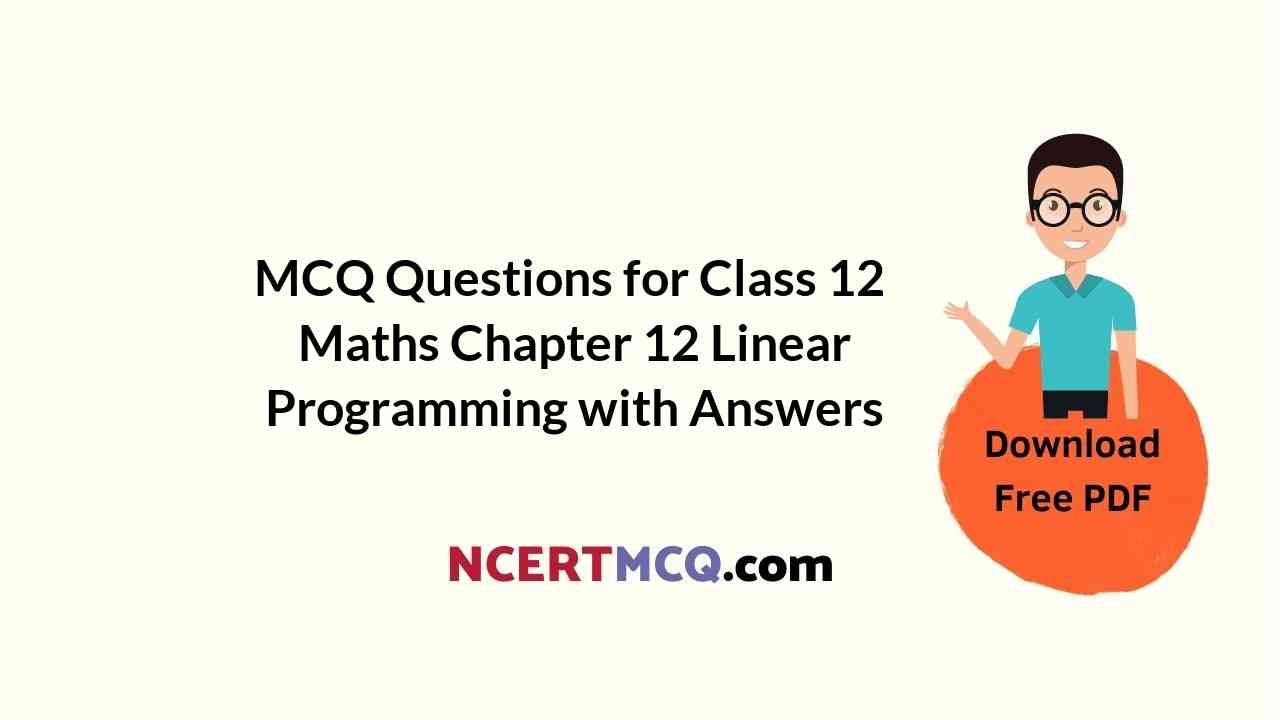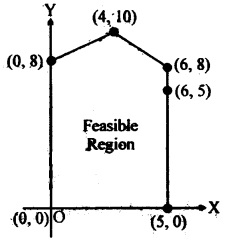Check the below NCERT MCQ Questions for Class 12 Maths Chapter 12 Linear Programming with Answers Pdf free download. MCQ Questions for Class 12 Maths with Answers were prepared based on the latest exam pattern. We have provided Linear Programming Class 12 Maths MCQs Questions with Answers to help students understand the concept very well.

## Linear Programming Class 12 MCQs Questions with Answers

Linear Programming Class 12 MCQ Question 1.
Feasible region in the set of points which satisfy
(a) The objective functions
(b) Some the given constraints
(c) All of the given constraints
(d) None of these

Answer: (c) All of the given constraints

MCQ Questions On Linear Programming Class 12 Question 2.
Of all the points of the feasible region for maximum or minimum of objective function the points
(a) Inside the feasible region
(b) At the boundary line of the feasible region
(c) Vertex point of the boundary of the feasible region
(d) None of these

Answer: (c) Vertex point of the boundary of the feasible region

Linear Programming MCQ With Answers Pdf Question 3.
Objective function of a linear programming problem is
(a) a constraint
(b) function to be obtimized
(c) A relation between the variables
(d) None of these

Answer: (b) function to be obtimized

Linear Programming MCQ Class 12 Question 4.
A set of values of decision variables which satisfies the linear constraints and nn-negativity conditions of a L.P.P. is called its
(a) Unbounded solution
(b) Optimum solution
(c) Feasible solution
(d) None of these

Lpp MCQ Class 12 Question 5.
The maximum value of the object function Z = 5x + 10 y subject to the constraints x + 2y ≤ 120, x + y ≥ 60, x – 2y ≥ 0, x ≥ 0, y ≥ 0 is
(a) 300
(b) 600
(c) 400
(d) 800

Lpp MCQ Questions Class 12 Question 6.
The maximum value of Z = 4x + 2y subject to the constraints 2x + 3y ≤ 18, x + y ≥ 10, x, y ≤ 0 is
(a) 36
(b) 40
(c) 30
(d) None of these

Lpp Class 12 MCQ Question 7.
In equation 3x – y ≥ 3 and 4x – 4y > 4
(a) Have solution for positive x and y
(b) Have no solution for positive x and y
(c) Have solution for all x
(d) Have solution for all y

Answer: (a) Have solution for positive x and y

Linear Programming Is A MCQ Question 8.
The maximum value of Z = 3x + 4y subjected to contraints x + y ≤ 40, x + 2y ≤ 60, x ≥ 0 and y ≥ 0 is
(a) 120
(b) 140
(c) 100
(d) 160

MCQ On Lpp Class 12 Question 9.
Maximize Z = 11 x + 8y subject to x ≤ 4, y ≤ 6, x + y ≤ 6, x ≥ 0, y ≥ 0.
(a) 44 at (4, 2)
(b) 60 at (4, 2)
(c) 62 at (4, 0)
(d) 48 at (4, 2)

Answer: (b) 60 at (4, 2)

MCQ Of Linear Programming Class 12 Question 10.
Maximize Z = 3x + 5y, subject to x + 4y ≤ 24, 3x + y ≤ 21, x + y ≤ 9, x ≥ 0, y ≥ 0
(a) 20 at (1, 0)
(b) 30 at (0, 6)
(c) 37 at (4, 5)
(d) 33 at (6, 3)

Answer: (c) 37 at (4, 5)

MCQ On Linear Programming Question 11.
Maximize Z = 4x + 6y, subject to 3x + 2y ≤ 12, x + y ≥ 4, x, y ≥ 0
(a) 16 at (4, 0)
(b) 24 at (0, 4)
(c) 24 at (6, 0)
(d) 36 at (0, 6)

Answer: (d) 36 at (0, 6)

MCQ On Linear Programming Class 12 Question 12.
Maximize Z = 7x + 11y, subject to 3x + 5y ≤ 26, 5x + 3y ≤ 30, x ≥ 0, y ≥ 0
(a) 59 at ($$\frac{9}{2}$$, $$\frac{5}{2}$$)
(b) 42 at (6, 0)
(c) 49 at (7, 0)
(d) 57.2 at (0, 5.2)

Answer: (a) 59 at ($$\frac{9}{2}$$, $$\frac{5}{2}$$)

MCQ Of Lpp Class 12 Question 13.
Maximize Z = 6x + 4y, subject to x ≤ 2, x + y ≤ 3, -2x + y ≤ 1, x ≥ 0, y ≥ 0
(a) 12 at (2, 0)
(b) $$\frac{140}{3}$$ at ($$\frac{2}{3}$$, $$\frac{1}{3}$$)
(c) 16 at (2, 1)
(d) 4 at (0, 1)

Answer: (c) 16 at (2, 1)

Class 12 Maths Chapter 12 MCQ Question 14.
Maximize Z = 10 x1 + 25 x2, subject to 0 ≤ x1 ≤ 3, 0 ≤ x2 ≤ 3, x1 + x2 ≤ 5
(a) 80 at (3, 2)
(b) 75 at (0, 3)
(c) 30 at (3, 0)
(d) 95 at (2, 3)

Answer: (d) 95 at (2, 3)

Linear Programming MCQs  Question 15.
Z = 20x1 + 202, subject to x1 ≥ 0, x2 ≥ 0, x1 + 2x2 ≥ 8, 3x1 + 2x2 ≥ 15, 5x1 + 2x2 ≥ 20. The minimum value of Z occurs at
(a) (8, 0)
(b) ($$\frac{5}{2}$$, $$\frac{15}{4}$$)
(c) ($$\frac{7}{2}$$, $$\frac{9}{4}$$)
(d) (0, 10)

Answer: (c) ($$\frac{7}{2}$$, $$\frac{9}{4}$$)

Linear Programming MCQ Class 12 Question 16.
Z = 6x + 21 y, subject to x + 2y ≥ 3, x + 4y ≥ 4, 3x + y ≥ 3, x ≥ 0, y ≥ 0. The minimum value of Z occurs at
(a) (4, 0)
(b) (28, 8)
(c) (2, $$\frac{7}{2}$$)
(d) (0, 3)

Answer: (c) (2, $$\frac{7}{2}$$)

Linear Programming Class 12 MCQ Questions Question 17.
The corner point of the feasible region determined by the system of linear constraints are (0, 0), (0, 40), (20, 40), (60, 20), (60, 0). The objective function is Z = 4x + 3y.
Compare the quantity in Column A and Column B

 Column A Column B Maximum of Z 325

(a) The quantity in column A is greater
(b) The quantity in column B is greater
(c) The two quantities are equal
(d) The relationship cannot be determined On the basis of the information supplied

Answer: (b) The quantity in column B is greater

Question 18.
The feasible region for a LPP is shown shaded in the figure. Let Z = 3x – 4y be the objective function. Minimum of Z occurs at(a) (0, 0)
(b) (0, 8)
(c) (5, 0)
(d) (4, 10)

Question 19.
Refer to Question 18 maximum of Z occurs at
(a) (5, 0)
(b) (6, 5)
(c) (6, 8)
(d) (4, 10)

Question 20.
Refer to Question 18 (Maximum value of Z+ Minimum value of Z) is equal to
(a) 13
(b) 1
(c) -13
(d) -17